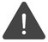# ^D

Access data as decimal or implied-decimal

 WTSupported in traditional Synergy on Windows WNSupported in Synergy .NET on Windows USupported on UNIX VSupported on OpenVMS
```^D(expression[, precision])
```

Arguments

expression

An expression to be accessed as decimal or implied-decimal. (a or n)

precision

(optional) The fractional precision. (n)

Discussion

^D accesses data as a decimal or implied-decimal data type.

^D does not convert expression to a decimal or implied-decimal value; the data itself remains unchanged. Only the data type is changed for the reference. (If you need to convert an expression to an implied-decimal value, use the %IMPLIED function. (See %IMPLIED.) There is no conversion function that creates a decimal from another type.)Before using ^D(expression) in another expression, be sure to validate that the data is numeric. Using ^D on non-ASCII numeric (for example, integer) data yields undefined numeric results.

If you specify precision, the expression is accessed as implied-decimal with the specified fractional precision. If you omit precision, the expression is accessed as decimal. Precision cannot be less than zero, greater than 28, or greater than the size of expression.

Examples

```record drec
len         ,d4
siz         ,d4
val         ,d4
record
amount      ,d10.4
proc
clear ^d(drec)                      ;Clear all decimals with zeros
amount = 234.5678
; ^d(amount, 2) changes amount from a d10.4 to a d10.2
; This causes the contents of amount to be viewed as 123456.78
^d(amount, 2) = %round(^d(amount, 2))   ;Round off last two digits
;Amount will contain 234.57
xreturn
end
```